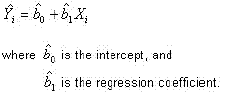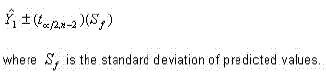### CFA Practice Question

There are 208 practice questions for this study session.

### CFA Practice Question

Which of the following statements is (are) true with respect to predicting the value of a dependent variable using a regression?

I. As the value of the independent variable increases, so will the value of the dependent variable.
II. Confidence interval around predicted values for the dependant variable will increase as the degrees of freedom increase.
III. The variance of predicted values is simply the square of the standard error of the estimate (SEE).
IV. The farther the independent variable is from its mean, the greater the dispersion will be around the predicted value for the dependent variable.
A. II and IV
B. I, II and III
C. IV
Explanation: A simple linear regression equation may be defined as follows:Therefore, if the regression coefficient were to be a negative value, then as the independent value increases, the dependent value would decrease further. The confidence interval around a predicted value for Y may be defined as follows:Therefore, as the degrees of freedom increase, the t-value will shrink; thus tightening the possible dispersion around the predicted value for Y.

The variance of predicted values may be computed as follows:Therefore, the farther Xi is from its mean, the greater (Xi - X-bar)2 will be, and thus the greater S2f will be. Consequently, the dispersion around the predicted value of Y will become greater.